# Lesson 2 2 Venn Diagrams and Logic Venn

• Slides: 31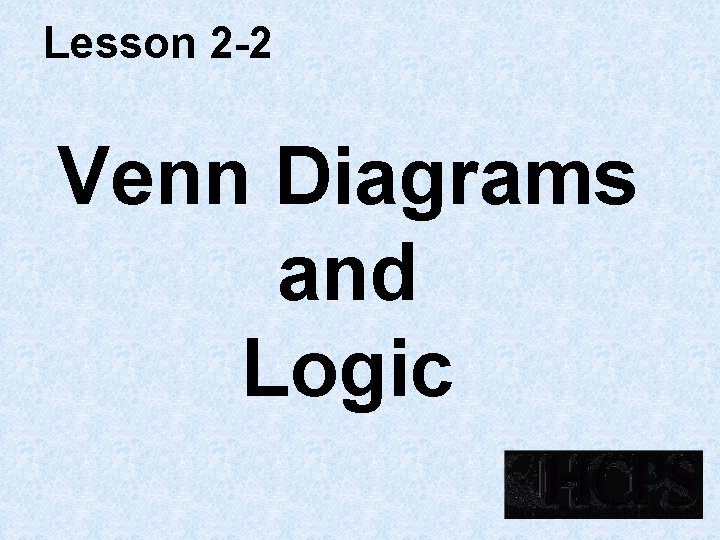Lesson 2 -2 Venn Diagrams and Logic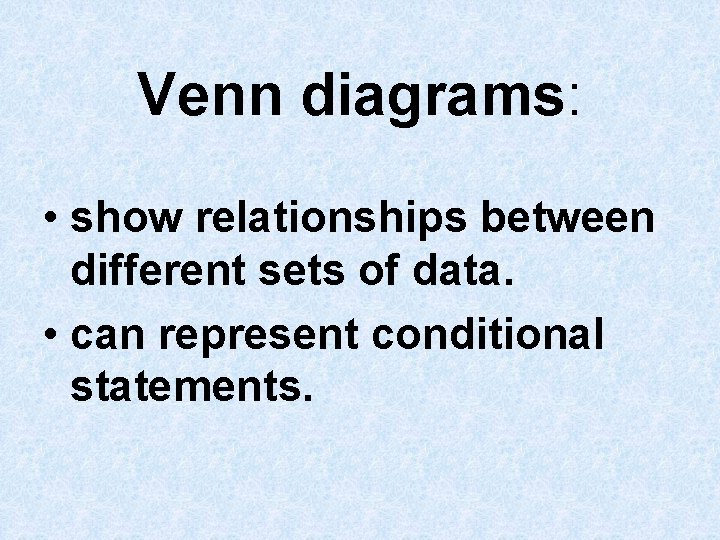Venn diagrams: • show relationships between different sets of data. • can represent conditional statements.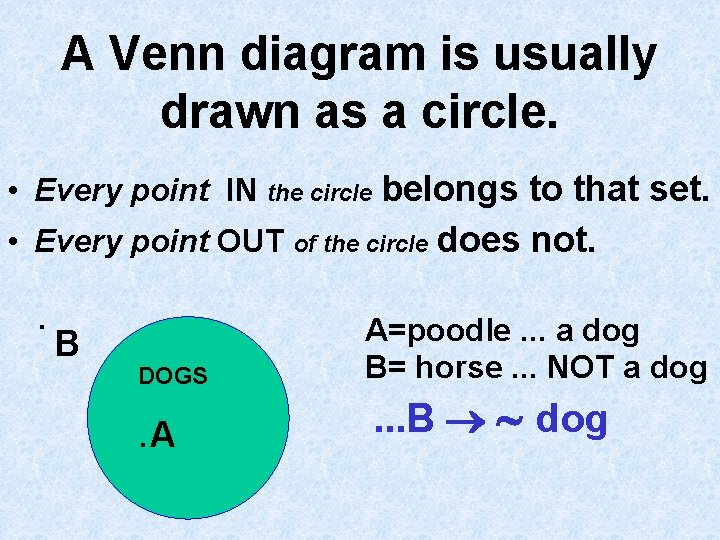A Venn diagram is usually drawn as a circle. • Every point IN the circle belongs to that set. • Every point OUT of the circle does not. . B DOGS . A A=poodle. . . a dog B= horse. . . NOT a dog . . . B dog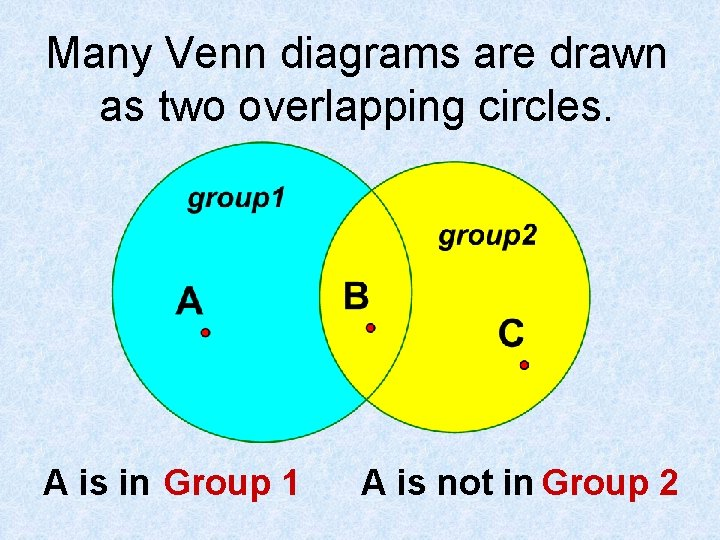Many Venn diagrams are drawn as two overlapping circles. A is in Group 1 A is not in Group 2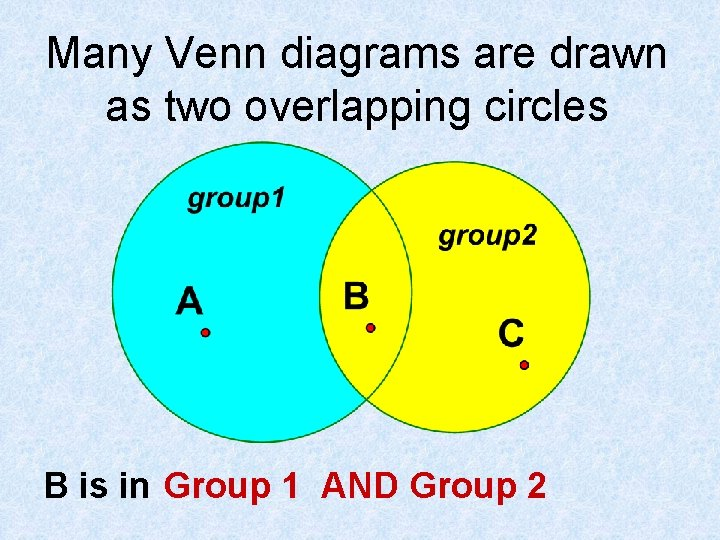Many Venn diagrams are drawn as two overlapping circles B is in Group 1 AND Group 2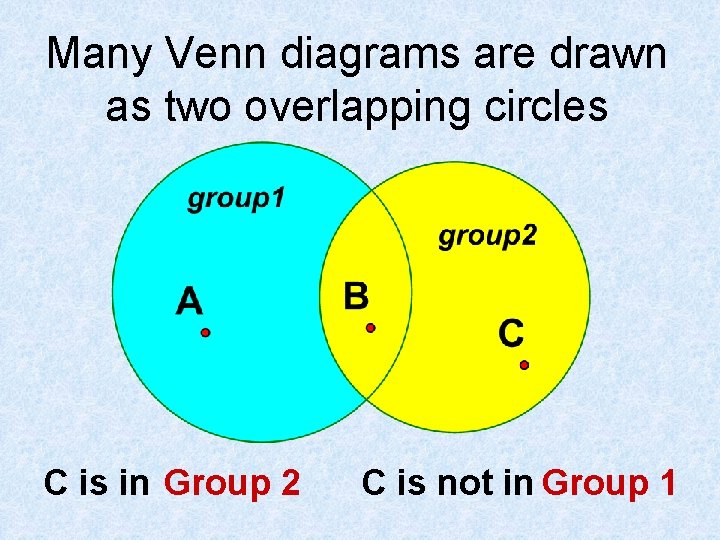Many Venn diagrams are drawn as two overlapping circles C is in Group 2 C is not in Group 1Many Venn diagrams are drawn as two overlapping circles Some of the elements in group 1 are in group 2 Some of the elements in group 2 are in group 1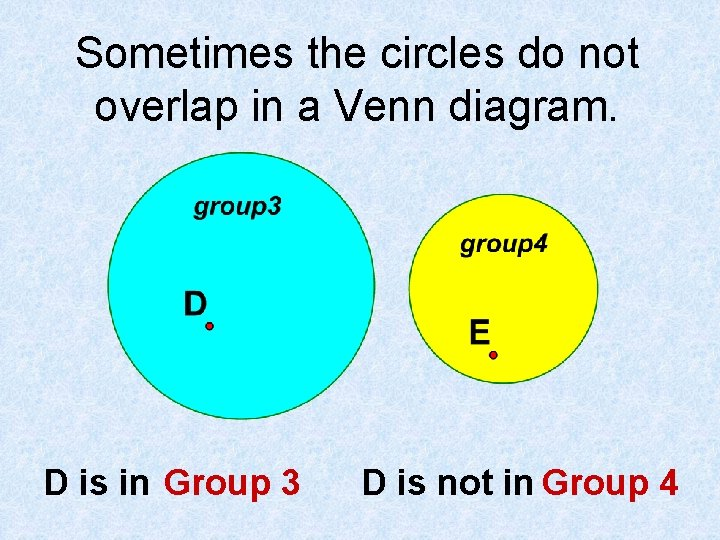Sometimes the circles do not overlap in a Venn diagram. D is in Group 3 D is not in Group 4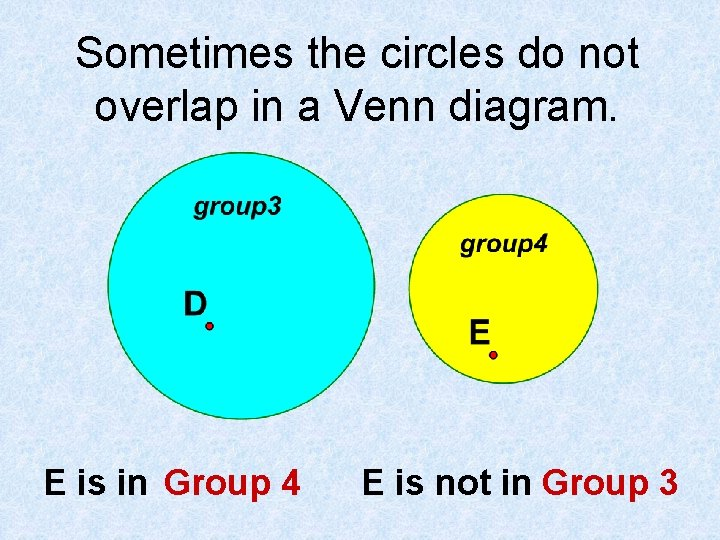Sometimes the circles do not overlap in a Venn diagram. E is in Group 4 E is not in Group 3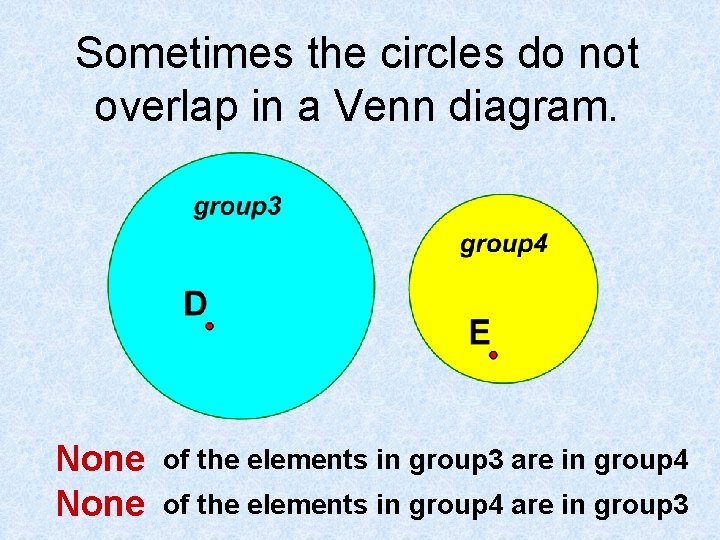Sometimes the circles do not overlap in a Venn diagram. None of the elements in group 3 are in group 4 of the elements in group 4 are in group 3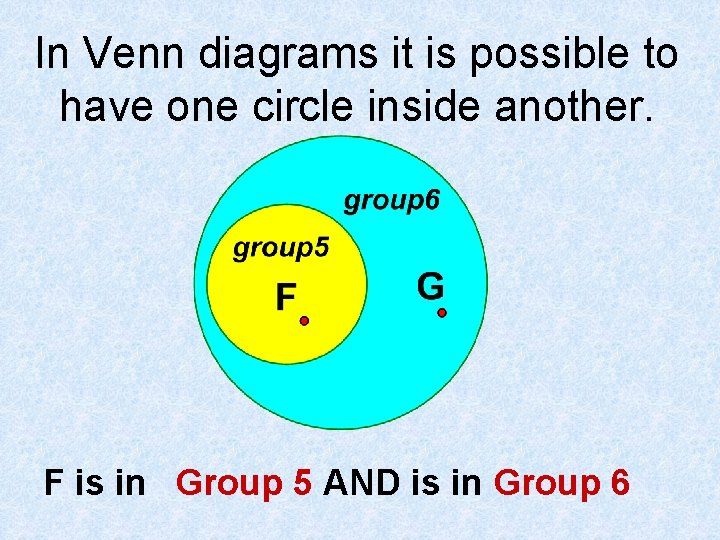In Venn diagrams it is possible to have one circle inside another. F is in Group 5 AND is in Group 6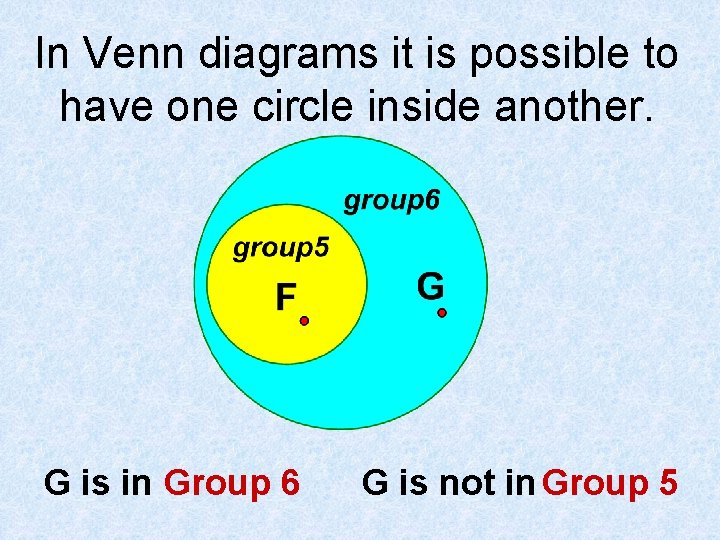In Venn diagrams it is possible to have one circle inside another. G is in Group 6 G is not in Group 5In Venn diagrams it is possible to have one circle inside another. of the elements in group 5 are in group 6 All Some of the elements in group 6 are in group 5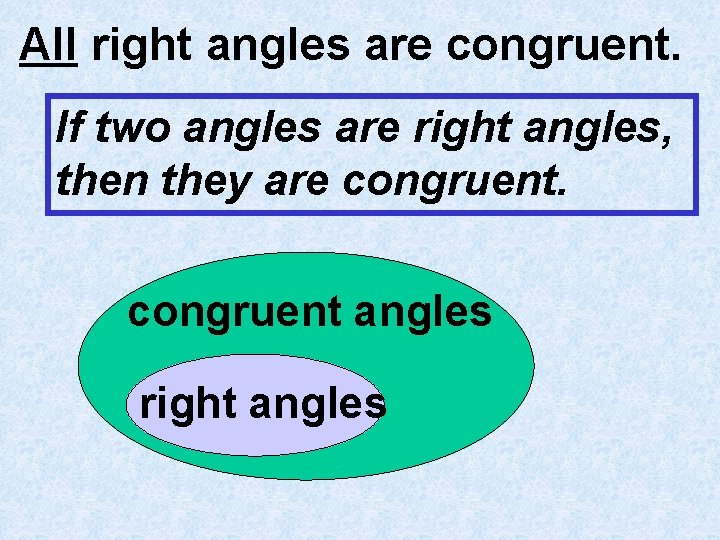All right angles are congruent. If two angles are right angles, then they are congruent angles right angles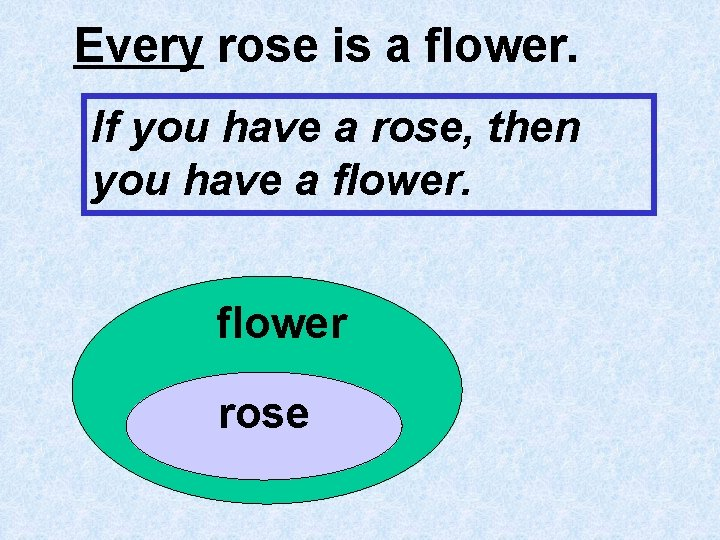Every rose is a flower. If you have a rose, then you have a flower roseIf two lines are parallel, then they do not intersect. Lines that do not intersect parallel lines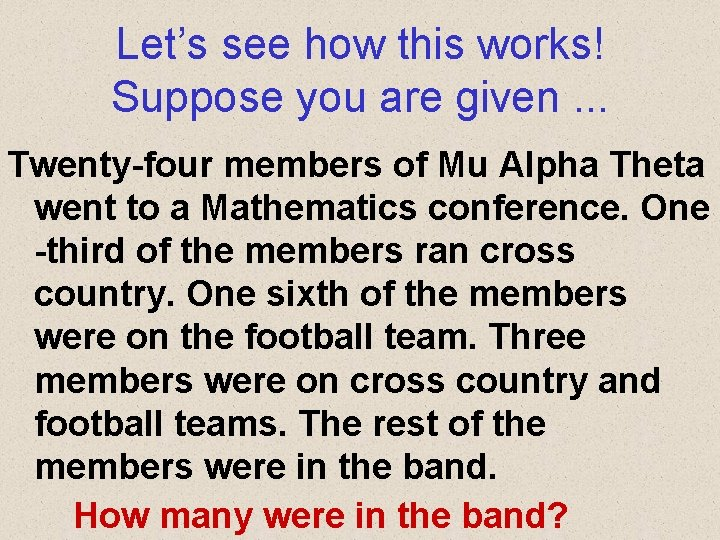Let’s see how this works! Suppose you are given. . . Twenty-four members of Mu Alpha Theta went to a Mathematics conference. One -third of the members ran cross country. One sixth of the members were on the football team. Three members were on cross country and football teams. The rest of the members were in the band. How many were in the band?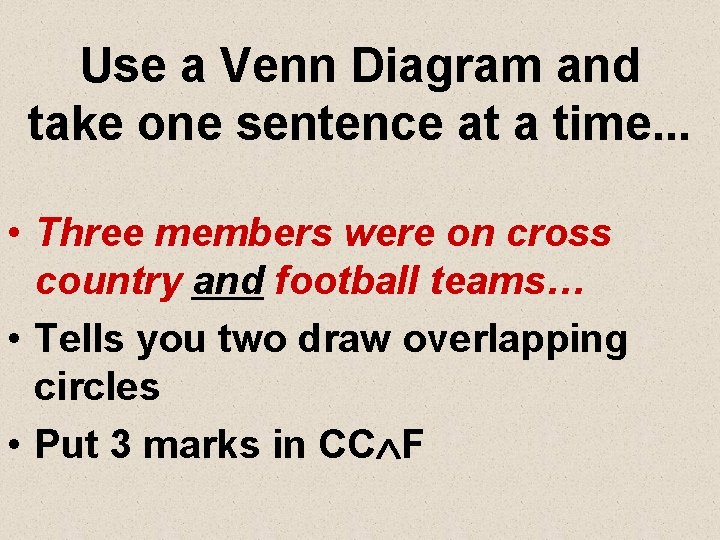Use a Venn Diagram and take one sentence at a time. . . • Three members were on cross country and football teams… • Tells you two draw overlapping circles • Put 3 marks in CC FUse a Venn Diagram and take one sentence at a time. . . • One-third of the members ran cross country. • put 8 marks in the CC circle since there are 24 members • already 3 marks • so put 5 marks in the red partUse a Venn Diagram and take one sentence at a time. . . • One sixth of the members were on the football team. • put 4 marks in the Football circle since there are 24 members • already 3 marks • so put 1 mark IIIII in the purple part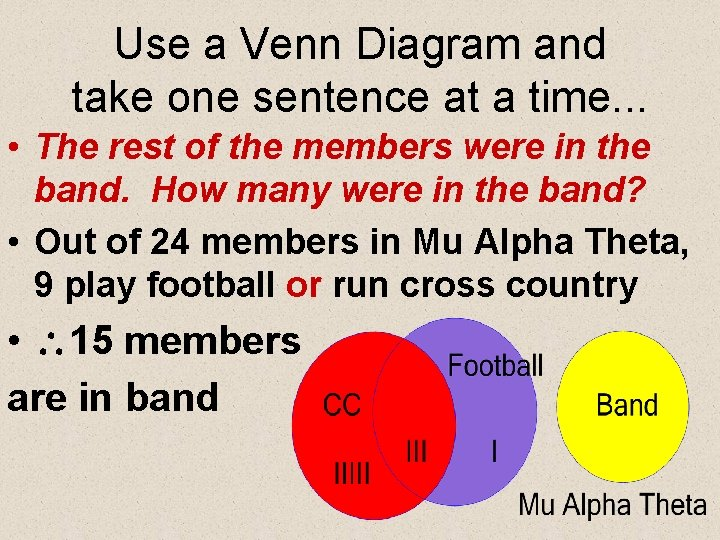Use a Venn Diagram and take one sentence at a time. . . • The rest of the members were in the band. How many were in the band? • Out of 24 members in Mu Alpha Theta, 9 play football or run cross country • 15 members are in band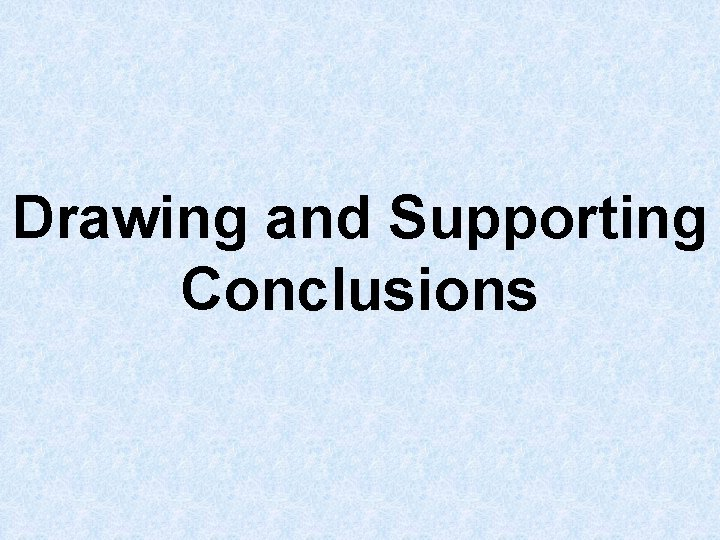Drawing and Supporting Conclusions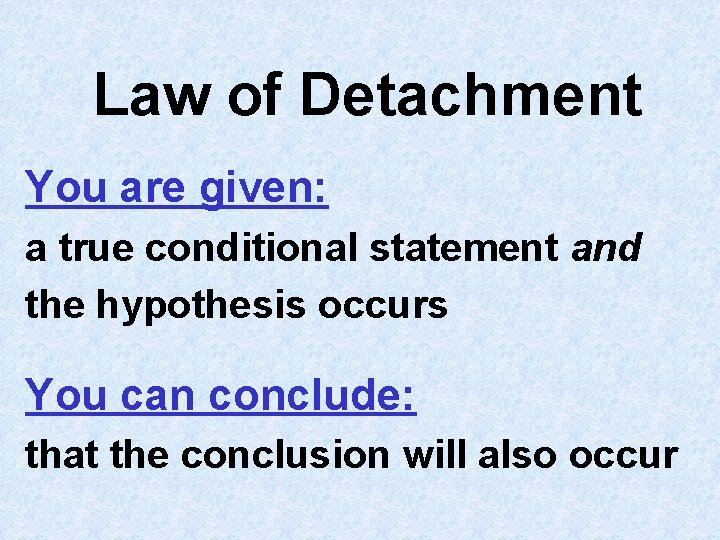Law of Detachment You are given: a true conditional statement and the hypothesis occurs You can conclude: that the conclusion will also occur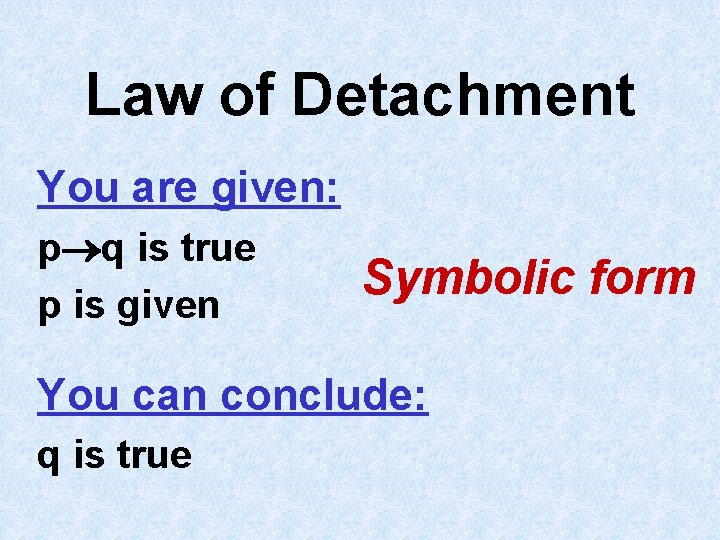Law of Detachment You are given: p q is true p is given Symbolic form You can conclude: q is trueLaw of Detachment Example You are given: If three points are collinear, then the points are all on one line. E, F, and G are collinear. You can conclude: E, F, and G are all on one line.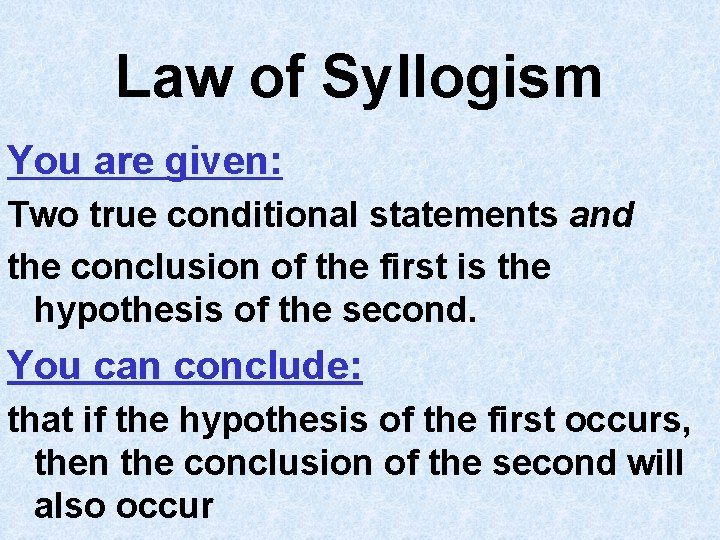Law of Syllogism You are given: Two true conditional statements and the conclusion of the first is the hypothesis of the second. You can conclude: that if the hypothesis of the first occurs, then the conclusion of the second will also occur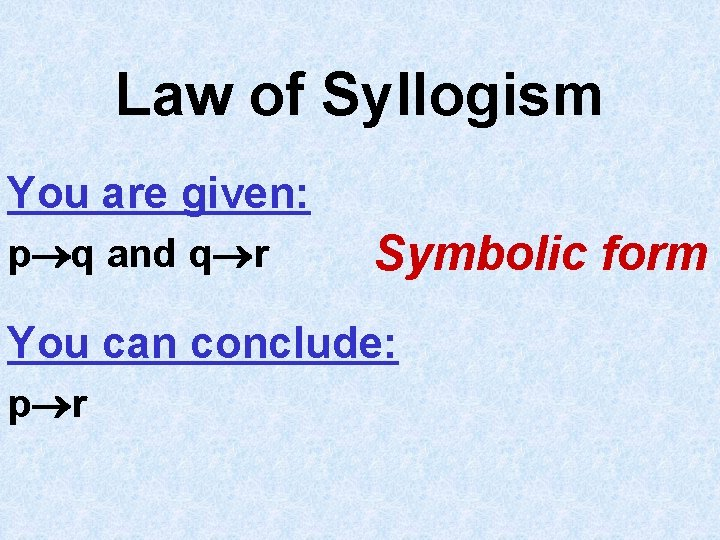Law of Syllogism You are given: p q and q r Symbolic form You can conclude: p rLaw of Syllogism You are given: Example If it rains today, then we will not have a picnic. If we do not have a picnic, then we will not see our friends. You can conclude: If it rains today, then we will not see our friends.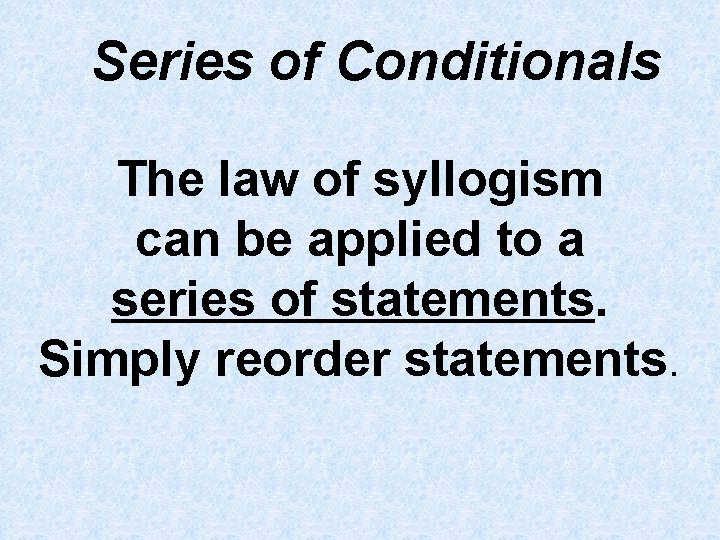Series of Conditionals The law of syllogism can be applied to a series of statements. Simply reorder statements.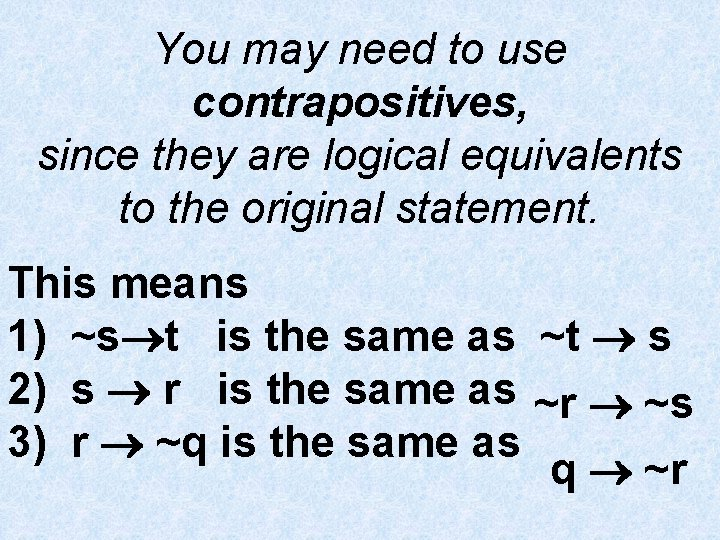You may need to use contrapositives, since they are logical equivalents to the original statement. This means 1) ~s t is the same as ~t s 2) s r is the same as ~r ~s 3) r ~q is the same as q ~rEXAMPLE: if p q, s r, ~s t, and r ~q; then p ______? You might need the contrapositives: ~t s or ~r ~s or q ~r Start with p and use the law of syllogism to find the conclusion: p q … q ~r … ~r ~s … ~s t p t